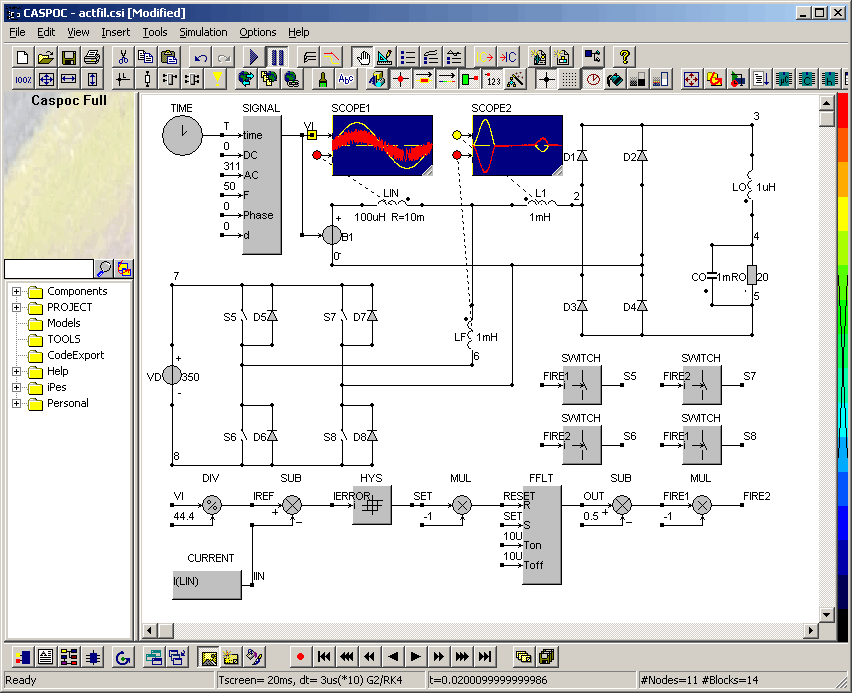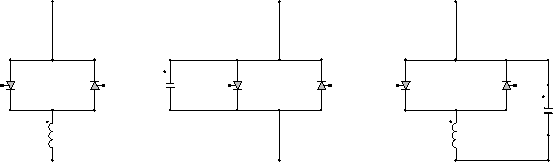• Introduction
• What is in this manual
• What is Caspoc
• User interface
• Introduction
• Starting
• Simulation
• Editing
• Viewing and printing
• Getting Started
• Basic editing
• Simulation in the time domain
• Basic User Interface Topics
• Editing
• Simulation
• Viewing
• Library
• Reports
• Project management
• Circuit and Block Diagram Components
• Introduction
• Cscript and user defined functions
• Component parameters
• Modeling Topics
• Introduction
• Power Electronics
• Semiconductors
• Electrical Machines
• Electrical drives
• Power Systems
• Mechanical Systems
• Thermal Systems
• Magnetic Circuits
• Green Energy
• Coupling to FEM
• Experimenter
• Analog hardware description language
• Embedded C code Export
• Coupling to Spice
• Small Signal Analysis
• Matlab coupling
• Tips and tricks
• Appendices

## Flexible AC Transmission Systems.

Flexible AC transmission systems (FACTS) are used in power systems for regulation and control. Based on semiconductor power electronics, FACTS are in actually high power power electronic circuits, such as rectifiers, inverters and controlled valves. The only difference is that they are combined with static reactive components such as inductors and capacitors and their combination leads to electric circuits that are used to control the power flow in a power system.

In Caspoc all basic components for building FACTS are available. There are electric circuit components like capacitors, inductors, diodes, thyristors, GTOs and IGBTs and the contro lcan be set up be making any type of analog or discrete control using the block diagram components. Special blocks are available in the library, for example the Components/Library/AdvancedElectricalDrives library houses a number of blocks in space vector format.

The simulation below shows a single phase active filter. Reachtive power is stored in the DC capacitive link and used to control the power factor at the input of the circuit. In this simulation the capacitive bufffer is replaced by a constant voltage source. Scope 1 shows the input voltage and current while scope 2 shows the input current of the rectfier and the input current from the compensator.Among the various FACTS the most aplied systems in use are

• Static Synchronous Compensator

• Static Synchronous Series Compensator

• Static Var Compensator

• Unified Power Flow Controller

Static Synchronous Compensator
The Static Synchronous Compensator (STATCOM) is a shunt device of the Flexible AC Transmission Systems (FACTS) family using power electronics to control power flow and improve transient stability on power grids. The STATCOM regulates voltage at its terminal by controlling the amount of reactive power injected into or absorbed from the power system. When system voltage is low, the STATCOM generates reactive power (STATCOM capacitive). When system voltage is high, it absorbs reactive power (STATCOM inductive).

Static Synchronous Series Compensator
The Static Synchronous Series Compensator (SSSC) is a series device of the Flexible AC Transmission Systems (FACTS) family using power electronics to control power flow and improve power oscillation damping on power grids. The SSSC injects a voltage Vs in series with the transmission line where it is connected.

Static Var Compensator
As the SSSC does not use any active power source, the injected voltage must stay in quadrature with line current. By varying the magnitude Vq of the injected voltage in quadrature with current, the SSSC performs the function of a variable reactance compensator, either capacitive or inductive.
The variation of injected voltage is performed by means of a Voltage-Sourced Converter (VSC) connected on the secondary side of a coupling transformer. The VSC uses forced-commutated power electronic devices (GTOs, IGBTs or IGCTs) to synthesize a voltage V_conv from a DC voltage source.
A capacitor connected on the DC side of the VSC acts as a DC voltage source. A small active power is drawn from the line to keep the capacitor charged and to provide transformer and VSC losses, so that the injected voltage Vs is practically90degrees out of phase with current I. In the control system block diagram Vd_conv and Vq_conv designate the components of converter voltage V_conv which are respectively in phase and in quadrature with current. Two VSC technologies can be used for the VSC:

• VSC using GTO-based square-wave inverters and special interconnection transformers. Typically four three-level inverters are used to build a 48-step voltage waveform. Special interconnection transformers are used to neutralize harmonics contained in the square waves generated by individual inverters. In this type of VSC, the fundamental component of voltage V_conv is proportional to the voltage Vdc. Therefore Vdc has to varied for controlling the injected voltage.

• VSC using IGBT-based PWM inverters. This type of inverter uses Pulse-Width Modulation (PWM) technique to synthesizeasinusoidal waveform from a DC voltage with a typical chopping frequency of a few kilohertz. Harmonics are cancelled by connecting filters at the AC side of the VSC. This type of VSC uses a fixed DC voltage Vdc. Voltage V_conv is varied by changing the modulation index of the PWM modulator.

The Static Var Compensator (SVC) is a shunt device of the Flexible AC Transmission Systems (FACTS) family using power electronics to control power flow and improve transient stability on power grids. The SVC regulates voltage at its terminals by controlling the amount of reactive power injected into or absorbed from the power system. When system voltage is low, the SVC generates reactive power (SVC capacitive). When system voltage is high, it absorbs reactive power (SVC inductive). The variation of reactive power is performed by switching three-phase capacitor banks and inductor banks connected on the secondary side of a coupling transformer. Each capacitor bank is switched on and off by three thyristor switches (Thyristor Switched Capacitor or TSC). Reactors are either switched on-off (Thyristor Switched Reactor or TSR) or phase-controlled (Thyristor Controlled Reactor or TCR).

Unified Power Flow Controller
The Unified Power Flow Controller (UPFC) is the most versatile member of the Flexible AC Transmission Systems (FACTS) family using power electronics to control power flow on power grids. The UPFC uses a combination of a shunt controller (STATCOM) and a series controller (SSSC) interconnected through a common DC bus

Thyristor Controlled Reactors
Using the circuit compoents such as the thyristor, GTO and capacitors and inductors, any type of Thyristor Controlled Reactor (TCR) can be build. Examples are:

• GCSC
GTO Controlled Series Capacitor

• TSSC
Thyristor Switched Series Capacitor

• TCSC
Thyristor Controlled Series Capacitor

• TCVR
Thyristor Control Voltage Regulator

• TCPAR
Thyristor Control Phase Angle Regulator

• TCBR
Thyristor Controlled Braking Resistor